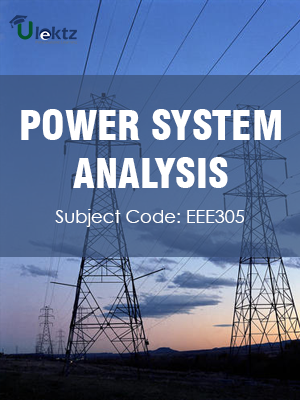•My WalletMy Order
•My Profile
•My Connections
•My Books
•My Videos
•My Tests
•My Calender
•My Messages
•My Shopping Cart
•My Orders
•Account Settings
•Help

# Book Details# Power System Analysis

 Course Code : EEE305 Author : Ulektz University : Kalasalingam University, Tamil Nadu Regulation : 2015 Categories : Electrical & Electronics Format :ePUB3 (DRM Protected) Type : eBook

FREE

Description :Power System Analysis of EEE305 covers the latest syllabus prescribed by Kalasalingam University, Tamil Nadu for regulation 2015. Author: Ulektz, Published by uLektz Learning Solutions Private Limited.

Note : No printed book. Only ebook. Access eBook using uLektz apps for Android, iOS and Windows Desktop PC.

##### Topics
###### UNIT 1: POWER SYSTEM MODELLING

1.1 Overview of power system analysis – importance of system planning and operational analysis, Matrix formation by building algorithm

1.2 Per phase analysis of symmetrical three phase system – modeling of power system components – representation, single line diagram, per unit representation

1.3 Network topology, Primitive network and its matrices

1.4 Bus admittance matrix formation – inspection method, singularity transformation method

1.5 Bus impedance

1.6 π equivalent circuit of transformer with off nominal – tap ratio – phase shifting transformer

###### UNIT 2: POWER FLOW ANALYSIS

2.1 Importance of power flow analysis– power flow problem

2.2 Classification of buses

2.3 Development of power flow model in Gauss-seidel power flow – numerical problems, Computation of transmission line flows, losses and slack bus power

2.4 Newton-Raphson (N-R) method (polar form)– flowchart – numerical problems

2.5 Development of Fast Decoupled Power Flow (FDPF) model, flowchart, numerical problems – comparison of the three methods of load flow

###### UNIT 3: SYMMETRIC FAULT ANALYSIS

3.1 Need for fault analysis - Common approximations made in fault analysis

3.2 Symmetrical short circuits – Thevenin‟s equivalent circuit and its applications

3.3 Short circuit capacity - Circuit breaker selections

3.4 Fault analysis using Z bus matrix

###### UNIT 4: UNSYMMETRICAL FAULT ANALYSIS

4.1 Unsymmetrical short circuits – short circuit analysis – symmetrical components method

4.2 Derivation of fault current – LG, LL, LLG short circuits – development of interconnection of sequence networks for LG, LL and LLG faults

###### UNIT 5: STABILITY ANALYSIS

5.1 Importance of stability analysis – classification of power system stability

5.2 Single Machine Infinite Bus (SMIB) system – development of swing equation – synchronous machine representation by classical model – power – angle equation– equal area criterion

5.3 Determination of critical clearing angle and time – algorithm for numerical solution of swing equation using modified Euler method – plotting of swing curves

### Related Books